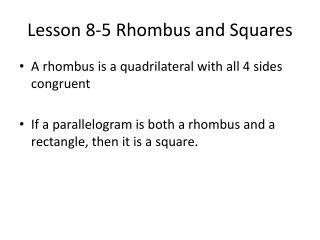# Lesson 8-5 Rhombus and Squares - PowerPoint PPT PresentationDownload PresentationLesson 8-5 Rhombus and Squares

Lesson 8-5 Rhombus and Squares
Download Presentation## Lesson 8-5 Rhombus and Squares

- - - - - - - - - - - - - - - - - - - - - - - - - - - E N D - - - - - - - - - - - - - - - - - - - - - - - - - - -
##### Presentation Transcript

1. Lesson 8-5 Rhombus and Squares • A rhombus is a quadrilateral with all 4 sides congruent • If a parallelogram is both a rhombus and a rectangle, then it is a square.

2. Rhombus Theorems • Theorem 8.15 The diagonals of a rhombus are perpendicular • Theorem 8.16 If the diagonals of a parallelogram are perpendicular, then the parallelogram is a rhombus • Theorem 8.17 Each diagonal of a rhombus bisects a pair of opposite angles

3. Given:BCDE is a rhombus, and Prove: D Example 5-1a

4. Proof: Because opposite angles of a rhombus are congruent and the diagonals of a rhombus bisect each other, By substitution, by the Reflexive Property and it is given that Therefore, by SAS. Example 5-1b

5. Given:ACDF is a rhombus; Prove: Example 5-1c

6. Proof: Since ACDF is a rhombus, diagonals bisect each other and are perpendicular to each other. Therefore, are both right angles. By definition of right angles, which means that by definition of congruent angles. It is given that so since alternate interior angles are congruent when parallel lines are cut by a transversal. by ASA. Example 5-1d

7. Use rhombus LMNP to find the value of y if N Example 5-2a

8. Example 5-2b The diagonals of a rhombus are perpendicular. Substitution Add 54 to each side. Take the square root of each side. Answer: The value of y can be 12 or –12.

9. Use rhombus LMNP to find if N Example 5-2c

10. Answer: Example 5-2d Opposite angles are congruent. Substitution The diagonals of a rhombus bisect the angles.

11. a. b. Answer: Example 5-2e Use rhombus ABCD and the given information to find the value of each variable. Answer: 8 or –8

12. Example 5-3a Determine whether parallelogram ABCD is a rhombus, a rectangle, or a square for A(–2, –1), B(–1, 3), C(3, 2), and D(2, –2). List all that apply. Explain. ExplorePlot the vertices on a coordinate plane.

13. Example 5-3a Plan If the diagonals are perpendicular, then ABCDis either a rhombus or a square. The diagonals of a rectangle are congruent. If the diagonals are congruent and perpendicular, then ABCD is a square. Solve Use the Distance Formula to compare the lengths of the diagonals.

14. Since the slope of is the negative reciprocal of the slope of the diagonals are perpendicular. The lengths of and are the same so the diagonals are congruent. ABCD is a rhombus, a rectangle, and a square. Example 5-3b Use slope to determine whether the diagonals are perpendicular.

15. Example 5-3c Examine The diagonals are congruent and perpendicular so ABCD must be a square. You can verify that ABCD is a rhombus by finding AB, BC, CD, AD. Then see if two consecutive segments are perpendicular. Answer: ABCD is a rhombus, a rectangle, and a square.

16. Example 5-3d Determine whether parallelogram EFGH is a rhombus, a rectangle, or a square for E(0, –2), F(–3, 0), G(–1, 3), and H(2, 1).List all that apply. Explain.

17. Answer: and slope of slope of Since the slope of is the negative reciprocal of the slope of , the diagonals are perpendicular. The lengths ofand are the same. Example 5-3d